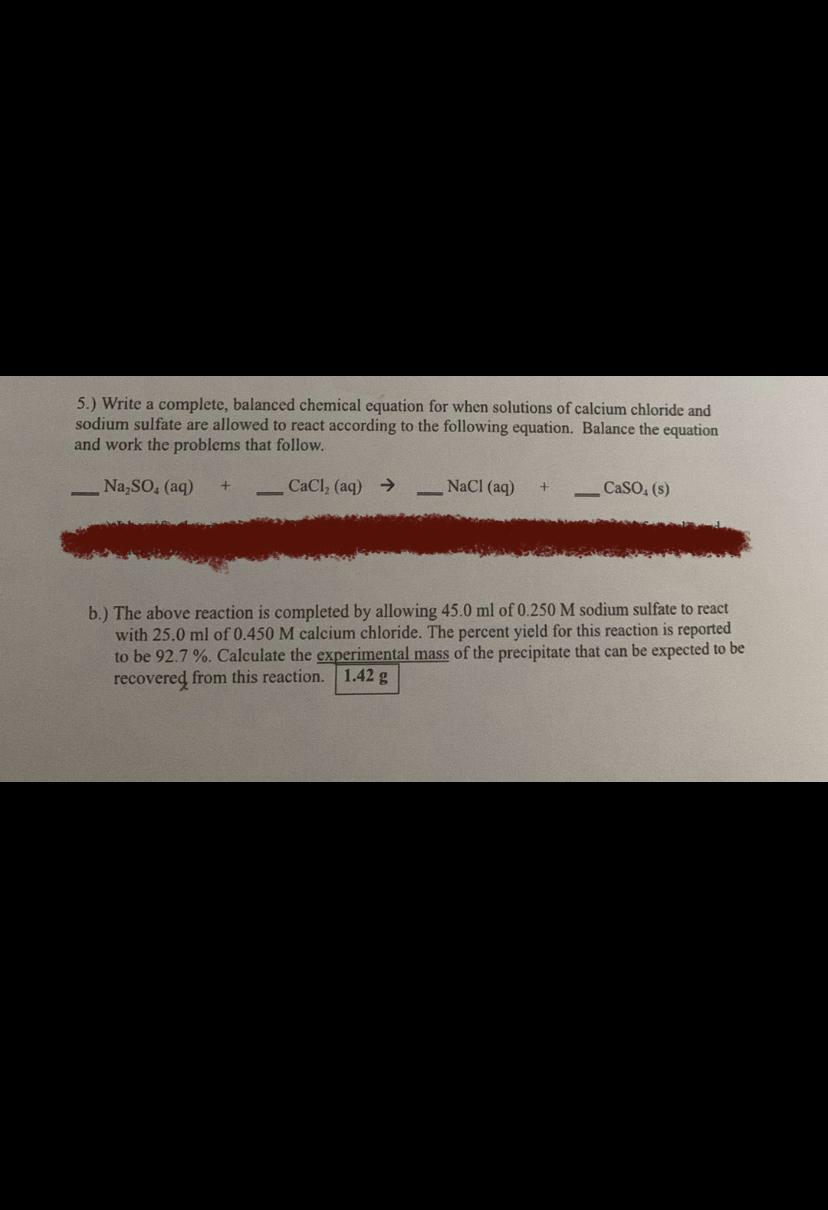Question:

# 5.) Write a complete, balanced chemical equation for when solutions of calcium chloride and sodium sulfate are allowed to react5.) Write a complete, balanced chemical equation for when solutions of calcium chloride and sodium sulfate are allowed to react according to the following equation. Balance the equation and work the problems that follow. Na So. (aq) CaCl, (aq) → NaCl(aq) CaSO. (5) b.) The above reaction is completed by allowing 45.0 ml of 0.250 M sodium sulfate to react with 25.0 ml of 0.450 M calcium chloride. The percent yield for this reaction is reported to be 92.7 %. Calculate the experimental mass of the precipitate that can be expected to be recovered from this reaction. 1.42 g